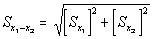‹ Consumption & Efficiency

# What is an RSE?

The estimates in the Commercial Buildings Energy Consumption Survey (CBECS) are based on data reported by representatives of a statistically-designed subset of the entire commercial building population in the United States, or a "sample". Consequently, the estimates differ from the true population values. However, the sample design permits us to estimate the sampling error in each value.

It is important to understand: CBECS estimates should not be considered as finite point estimates, but as estimates with some associated error in each direction.

The standard error is a measure of the reliability or precision of the survey statistic. The value for the standard error can be used to construct confidence intervals and to perform hypothesis tests by standard statistical methods. Relative Standard Error (RSE) is defined as the standard error (square root of the variance) of a survey estimate, divided by the survey estimate and multiplied by 100.

The 95-percent confidence range for a given survey estimate can be determined with the RSE. To calculate the 95-percent confidence range:

1. Divide the RSE by 100 and multiply by the survey estimate to determine the standard error.
2. Multiply the standard error by 1.96 to determine the confidence error.
3. The survey estimate plus or minus the confidence error is the 95-percent confidence range.

For example, from Table A1, the estimate for total floorspace for all commercial buildings in the 2003 CBECS is 71,658 square feet and the estimate's RSE is 3.1 percent. The standard error is (3.1/100)*(71,658 million square feet) or 2,221 million square feet. The 95-percent confidence error is (1.96)*(2,221 million square feet), or 4,354 million square feet. Therefore, with 95 percent confidence, the true amount of floorspace in commercial buildings in the U.S. in 2003 was 71,658 plus or minus 4,354 million square feet, or, the range was from 67,304 to 76,012 million square feet.

## Statistical Significance Between Two Statistics

The difference between any two estimates given in the Detailed Tables may or may not be statistically significant. Statistical significance is computed as:where S is the standard error, x1 is the first estimate, and x2 is the second estimate. The result of this computation is to be multiplied by 1.96 and, if this result is less than the difference between the two estimates, the difference is statistically significant.

For example, from Table B1, food sales buildings were an average 5,600 square feet in 2003 with an RSE of 7.5, while health care buildings averaged 24,600 square feet with an RSE of 11.4, for an estimated difference of 19,000 square feet between the two building types. The standard error for food sales buildings (x1) is (7.5/100)*5,600, or 420, and the standard error for health care buildings (x2) is (11.4/100)*24,600, or 2,804. So,

Sx1-x2 = (4202+2,8042)½

and

Sx1-x2 = 2,836.

Multiplying 2,836 by 1.96 yields 5,558. Since 5,558 is less than 19,000, the difference between the two estimates is statistically significant.

CBECS Survey Forms

CBECS Maps

CBECS Terminology

Survey Background & Technical Information

Building Type Definitions

Publications & Reports

#### Can I get this information by state?

See all CBECS FAQs ›

#### Features

2018 Consumption and Expenditures Highlights

2018 Building Characteristics Highlights

#### Other End Use Surveys

Manufacturing - MECS

Transportation